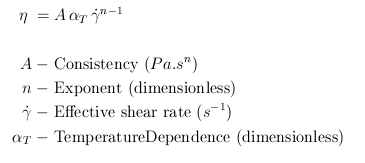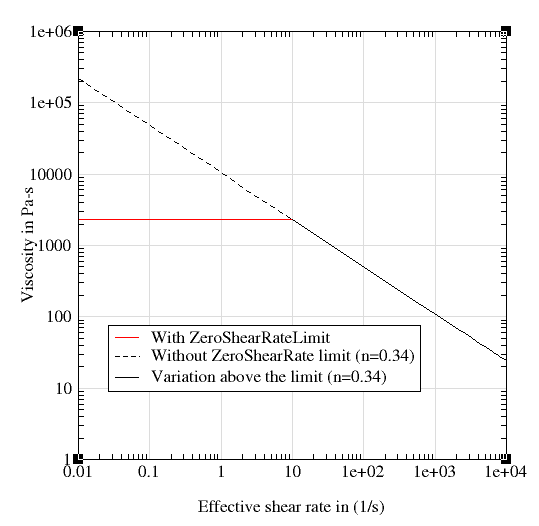﻿ Power Law Model
Manufacturing Solutions

# Power Law Model# Power Law ModelThis is a two parameter model and it is described by the following equation.This model describes the viscosity using a power-law relationship. The exponent n determines the nature of the relationship.

n < 1        Shear thinning or Pseudoplastic

n > 1        Shear thickening or dilalatant

n = 1        Newtonian

For most polymers, Exponent n is less than 1. When the exponent is less than 1, viscosity of the polymer decreases with increase in the shear rate. This behavior is called shear thinning. On the other hand, when the exponent is greater than 1, viscosity increases with shear rate and this behavior is called shear thickening. The following figure shows viscosity as a function of exponent (consistency = 1.05e+04)One of the disadvantages of the power-law model is, as the shear rate tends to zero (when the exponent is less than 1) the viscosity tends to infinity. This setback is overcome in HyperXtrude by defining a ZeroShearRateLimit. Typically, this value is chosen based on the experimental data used to determine the model coefficients. For instance, if the ZeroShearRateLimit is set to 10.0, then HyperXtrude will use this value to compute the viscosity whenever the effective shear rate of the flow field is less than the ZeroShearRateLimit. The following figure shows this effect for a power-law model with consistency 1.05e+04 and exponent 0.34.In addition to this, a few additional parameters arise due the temperature dependence of material data; these variables are discussed in Temperature Dependence.

Syntax

Syntax of the data packet Polymer is as follows:

 Polymer PolymerName { ConstitutuveModel = "PowerLaw" Density = ρ SpecificHeat = Cp(T) Conductivity = K(T) CoeffOfThermalExpansion = βT VolumetricHeatSource = Qvol Consistency = A Exponent = n ZeroShearRate = γ0 TemperatureDependence = "None" }

### Typical Value

ConstitutiveModel

Describes the model used

None

String

Required

"PowerLaw"

Density

Density of the polymer

kg/m^3

Constant

Required

995.0

SpecificHeat

Specific heat at constant pressure

J/kg/K

Constant / F(T)

Required

2000.0

Conductivity

Thermal conductivity

W/m/K

Constant / F(T)

Required

0.167

CoeffOfThermalExpansion

Indicates the change in volume with change in temperature

1/K

Constant

Required

1.0e-05

VolumetricHeatSource

Heat generated/ removed in the volume by methods like electrical heating

W/m^3

Constant

Required

0.0

Consistency

One of the parameters of the power law model. When n=1 it is same as viscosity.

Pa s^n

Constant

Required

1.0e+04

Exponent

Power law index, defines the dependency of viscosity on shear rate.

None

Constant

Required

0.66

ZeroShearRateLimit

See above

1/s

Constant

Required

0.01

TemperatureDependence

None

String

Required

"Exp(-Beta(DeltaT))"

ReferenceTemperature

Temperature at which data is calculated for the initialization step.

K

Constant

Required only if TD is not "None"

533

FreezeTemperature

This is the no flow temperature. Below this temperature, material ceases to flow.

K

Constant

Required only if TD is not "None"

350

ActivationEnergy

A parameter required by Arrhenius model.

J/mol

Constant

Required only if TD is Exp(Q/RT)

16628

UniversalGasConstant

A parameter from state equation PV = nRT, R is universal Gas constant.

J/mol/K

Constant

Required only if TD is Exp(Q/RT)

8.314

TemperatureSensitivity

A derived parameter which has the same physical meaning as Q/R.

K

Constant

Required only if TD is Exp(Tb/T)

2000 K

WLFConstant1

Constant C1 of WLF model

None

Constant

Required only if TD is WLF

17.44

WLFConstant2

Constant C2 of WLF model. This is like DeltaT, hence the value is same in K and Celsius.

K

Constant

Required only if TD is WLF

51.6

GlassTransitionTemperature

Temperature below with polymer molecules ceases to move (frozen). There are few definitions of this term.

K

Constant

Required only if TD is WLF

320

Beta

Parameter in the relationship Exp(-Beta(DeltaT))

None

Constant

Required only if TD is Exp(-Beta(DeltaT))

0.005

F(T) - Function of Temperature. Can be specified as a TABLE1 or TCL function.

TD - TemperatureDependence